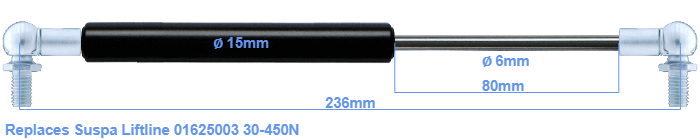# Replacement for Suspa Liftline 01625003 30-450N

\$31.35

Replacement gas spring for the Suspa Liftline 01625003 30-450 Newton. This fits a (possibly already present) ball with a diameter of 10mm. A corresponding ball is included. Brand: Stabilus Industry Line.
 Force Choose an option30 Newton40 Newton50 Newton60 Newton80 Newton100 Newton120 Newton140 Newton150 Newton160 Newton180 Newton200 Newton220 Newton240 Newton250 Newton260 Newton280 Newton300 Newton320 Newton340 Newton350 Newton360 Newton380 Newton400 Newton420 Newton440 Newton450 NewtonClear
This gas spring is also known as 16-1 016 25003, 16-1-113-84-A246-B246.The bore of the house of this replacement gas spring is 15 mm. The diameter of the rod is 6 millimeter. The rod (the chrome part) is 80 mm long. In total, the length is 236 millimeter. Keep in mind that this is the length between the centers of the mounting parts. The length from thread to thread (exlcuding the mounting parts) is 192 millimeter. The force of this replacement gas spring is 30-450 Newton. This gas spring can replace a Suspa Liftline gas spring, but is not an orignal Suspa Liftline one. This is a Stabilus Industry Line gas spring. The force (30-450 N) and dimensions are identical to the original.
Category: